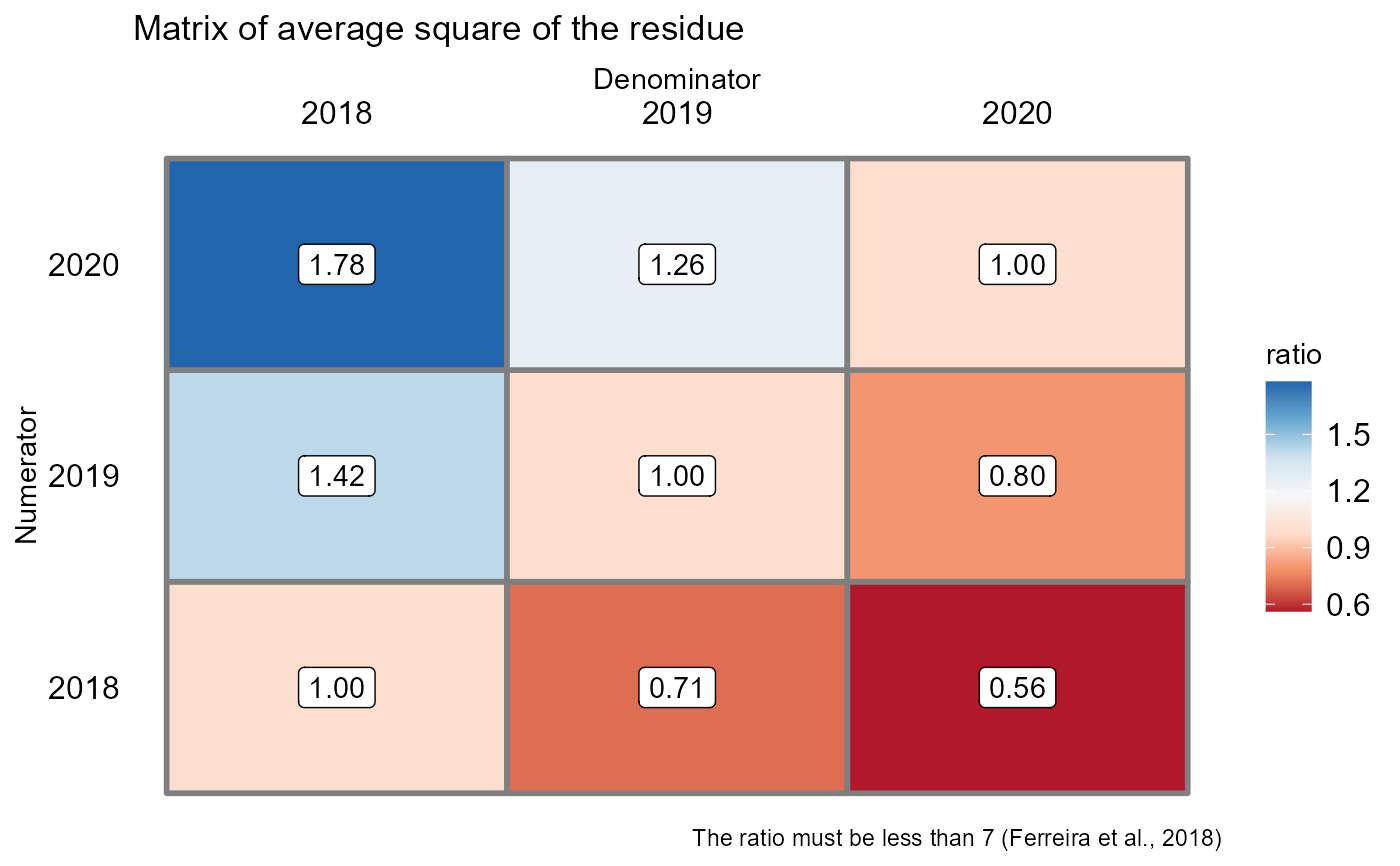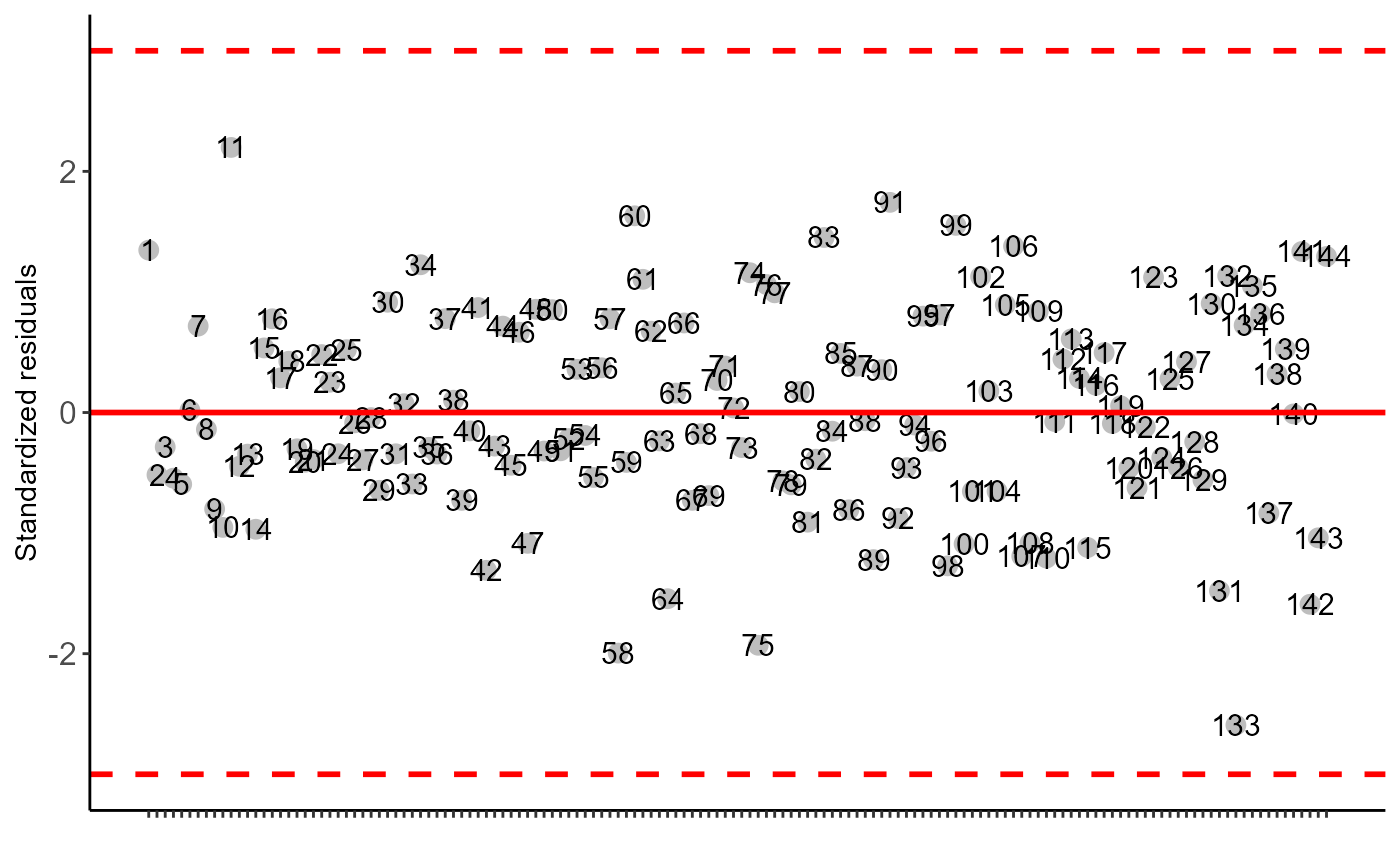Function of the AgroR package for joint analysis of experiments conducted in a randomized factorial double in block design with balanced data. The function generates the joint analysis through two models. Model 1: F-test of the effects of Factor 1, Factor 2 and F1 x F2 interaction are used in reference to the mean square of the interaction with the year. Model 2: F-test of the Factor 1, Factor 2 and F1 x F2 interaction effects are used in reference to the mean square of the residual.

conjfat2dbc(
f1,
f2,
block,
experiment,
response,
transf = 1,
constant = 0,
model = 1,
norm = "sw",
homog = "bt",
homog.value = 7,
alpha.f = 0.05,
alpha.t = 0.05
)

## Arguments

f1

Numeric or complex vector with factor 1 levels

f2

Numeric or complex vector with factor 2 levels

block

Numerical or complex vector with blocks

experiment

Numeric or complex vector with locations or times

response

Numerical vector containing the response of the experiment.

transf

Applies data transformation (default is 1; for log consider 0)

constant

Add a constant for transformation (enter value)

model

Define model of the analysis of variance

norm

Error normality test (default is Shapiro-Wilk)

homog

Homogeneity test of variances (default is Bartlett)

homog.value

Reference value for homogeneity of experiments. By default, this ratio should not be greater than 7

alpha.f

Level of significance of the F test (default is 0.05)

alpha.t

Significance level of the multiple comparison test (default is 0.05)

## Value

Returns the assumptions of the analysis of variance, the assumption of the joint analysis by means of a QMres ratio matrix and analysis of variance

## Note

The function is still limited to analysis of variance and assumptions only.

## Author

Gabriel Danilo Shimizu, shimizu@uel.br

Leandro Simoes Azeredo Goncalves

Rodrigo Yudi Palhaci Marubayashi

## Examples

library(AgroR)
ano=factor(rep(c(2018,2019,2020),e=48))
f1=rep(rep(c("A","B","C"),e=16),3)
f2=rep(rep(rep(c("a1","a2","a3","a4"),e=4),3),3)
resp=rnorm(48*3,10,1)
bloco=rep(c("b1","b2","b3","b4"),36)
#>
#> -----------------------------------------------------------------
#> Normality of errors
#> -----------------------------------------------------------------
#>                          Method Statistic   p.value
#>  Shapiro-Wilk normality test(W) 0.9936686 0.7794519
#>
#> As the calculated p-value is greater than the 5% significance level, hypothesis H0 is not rejected. Therefore, errors can be considered normal
#> -----------------------------------------------------------------
#> Homogeneity of Variances
#> -----------------------------------------------------------------
#>                               Method Statistic   p.value
#>  Bartlett test(Bartlett's K-squared)  23.44848 0.0152697
#>
#> As the calculated p-value is less than the 5% significance level, H0 is rejected. Therefore, the variances are not homogeneous
#>
#> -----------------------------------------------------------------
#> Independence from errors
#> -----------------------------------------------------------------
#>                  Method Statistic   p.value
#>  Durbin-Watson test(DW)  2.568423 0.8143241
#>
#> As the calculated p-value is greater than the 5% significance level, hypothesis H0 is not rejected. Therefore, errors can be considered independent
#> -----------------------------------------------------------------
#> Test Homogeneity of experiments
#> -----------------------------------------------------------------
#>  1.779032
#>
#> Based on the analysis of variance and homogeneity of experiments, it can be concluded that:
#> The experiments can be analyzed together#>
#>#>
#> -----------------------------------------------------------------
#> Analysis of variance
#> -----------------------------------------------------------------
#>             Df    Sum Sq   Mean Sq   F value    Pr(>F)
#> f1           2  1.588736 0.7943681 0.6529516 0.5303031
#> f2           3  8.457173 2.8190575 2.3171980 0.1035156
#> f1:f2        6  8.344925 1.3908208 1.1432215 0.3710006
#> local:bloco 11 12.026048 1.0932771 0.8986477 0.5563127
#> f1:f2:local 22 26.764767 1.2165803 1.2587297 0.1270165
#> Residuals   99 95.684923 0.9665144
#>
#> -----------------------------------------------------------------
#>